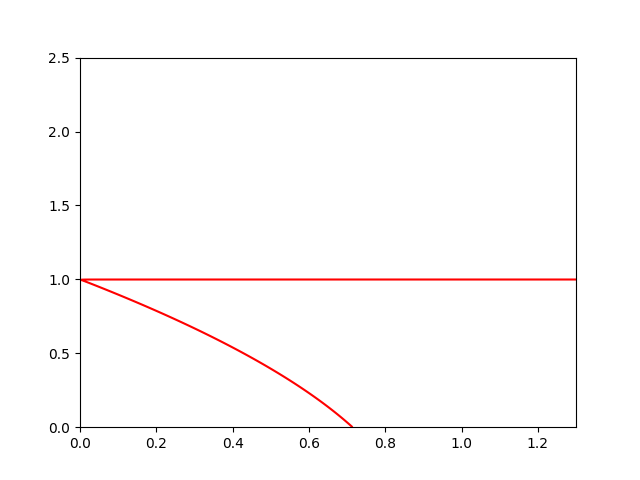# Avoid discontinuities in contour plot

Hi
I am using contour plot but I am facing the issue that it is plotting the points where the function is undefined. For example, I am using a rational function and it has a discontinuity in y = 1(red horizontal line.Is there any way to avoid this?

import matplotlib.pyplot as plt
import numpy as np

k=2.5

def R(x,y):
return 1-((x*(k+1))/((y+k)*(1-y)))

x = np.linspace(0,1.3,500)
y = np.linspace(0,2.5,500)

X, Y = np.meshgrid (x, y)

plt.contour(X, Y, R(X, Y), , colors=‘r’)

plt.show()

Contouring assumes your data is continuous, it has no way of knowing what you intend between grid points. If you

``````print(z[198:202, 40])
``````

you get

``````[-12.02984218 -33.69646609  52.9702432   15.82742857]
``````

so your `R` values go from negative to positive as they cross `y=1` and hence a contour line is drawn for `R=0`.

In this example you can get away with specifying one of your `y` points being exactly at 1 using

``````y = 1.0
``````

and hence your calculated `R` is infinite and a contour line is not drawn. But this is not generally reliable as you are at the mercy of finite floating-point precision.